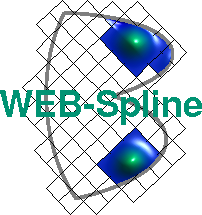# Error Estimates

To illustrate the WEB-method in an elementary setting, we consider a simple model problem, which has an analytic solution and, thus, permits the computation of exact approximation errors.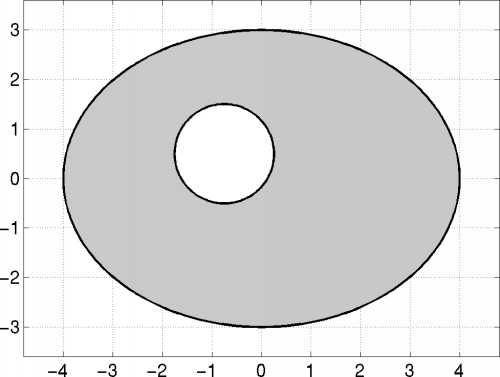The simulation domain, shown above, is bounded by an ellipse and a circle.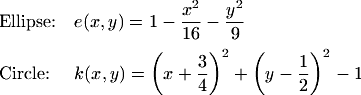Numerical solutions are computed for Poisson's equation with homogeneous Dirichlet boundary conditions.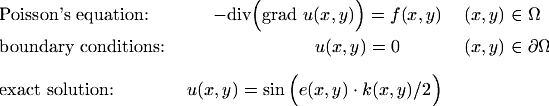The right-hand side is chosen from a given exact solution, which is depicted below.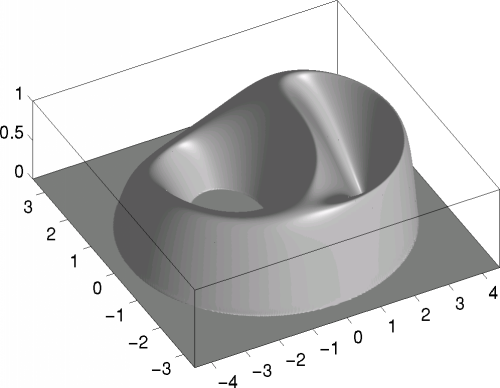For the construction of the WEB-basis we used the analytic weight function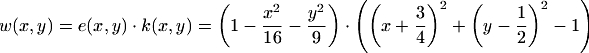The error was measured in the following two norms.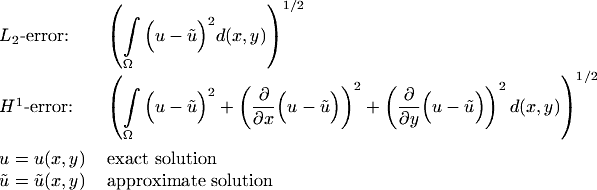For B-splines of order 2 to 6 (polynomial degree 1 to 5)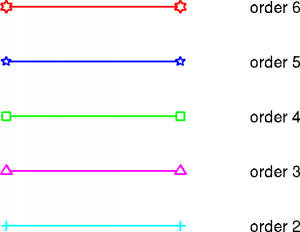the grid width was succesively halfed and the Ritz-Galerkin approximations computed. In the following diagram the L2-error was plotted versus the number of basis functions.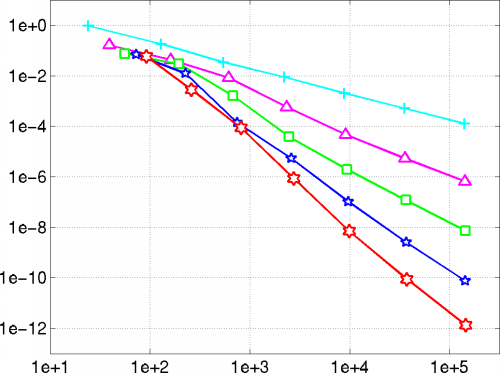Dividing consecutive errors for a fixed order and taking logarithms with respect to the base 2 yields estimates for the convergence rate. The diagram below confirms that the optimal rates are attained.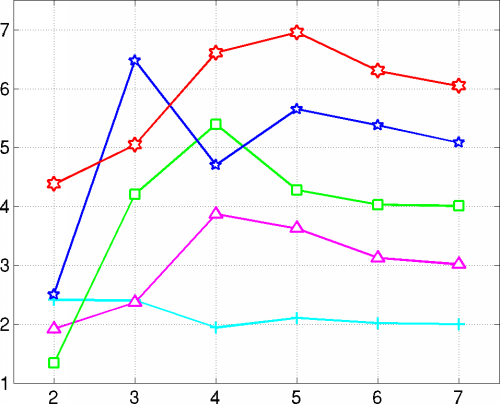The H1-norm corresponds to the natural energy norm associated with Poisson's operator.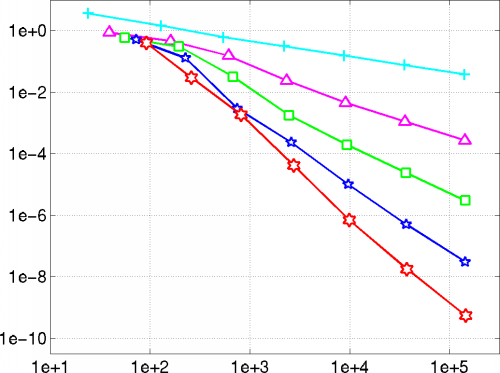The optimal approximation order is equal to the degree of the WEB-splines (order minus 1). Again, the numerical results correspond to the theoretical predictions. The qualitative behavior of the error is similar as for the L2-norm.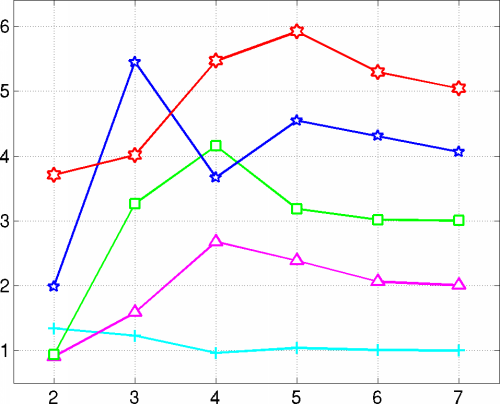The following diagram compares the L2-error for WEB-splines to that of linear trial functions on a triangulation (black caro markers).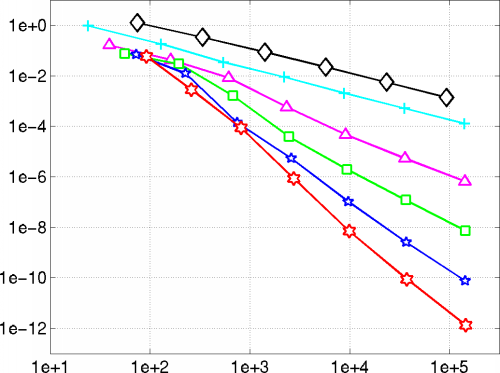Because of the stabilization of the B-splines, the Ritz-Galerkin system can be solved efficiently with standard iterative methods, such as the preconditioned conjugate gradient algorithm. The following diagram shows the number of pcg-iterations for a tolerance of 1e-14 as a function of the dimension of the system.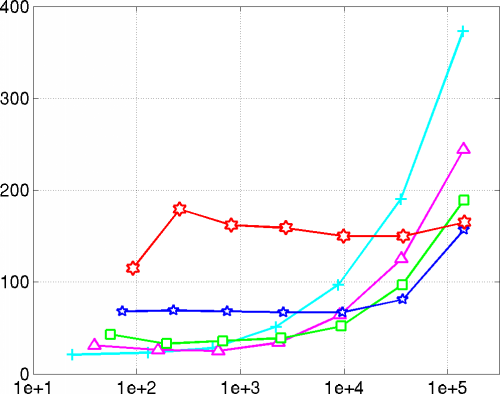### Literature

K. Höllig, U. Reif, J. Wipper: Weighted extended b-spline approximation of Dirichlet problems. SIAM Journal on Numerical Analysis, Volume 39, Number 2, pp. 442-462, 2001.

See Publications for a complete list of WEB-publications.

Author: Joachim Wipper ; Last modification: 2006/08/31 09:36:15 UTC.

 [Home] [The WEB-Method] [Examples] [Publications] [Contact]